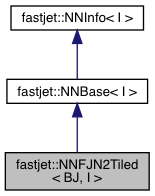FastJet  3.3.1
fastjet::NNFJN2Tiled< BJ, I > Class Template Reference

Helps solve closest pair problems with factorised interparticle and beam distances (ie satisfying the FastJet lemma) that are on a cylindrical geometry and allow tiling. More...

`#include <fastjet/NNFJN2Tiled.hh>`

Inheritance diagram for fastjet::NNFJN2Tiled< BJ, I >:[legend]
Collaboration diagram for fastjet::NNFJN2Tiled< BJ, I >:[legend]

## Public Member Functions

NNFJN2Tiled (const std::vector< PseudoJet > &jets, double requested_tile_size)
constructor with an initial set of jets (which will be assigned indices `0...jets.size()-1`)

NNFJN2Tiled (const std::vector< PseudoJet > &jets, double requested_tile_size, I *info)

void start (const std::vector< PseudoJet > &jets)
initialisation from a given list of particles

double dij_min (int &iA, int &iB)
return the dij_min and indices iA, iB, for the corresponding jets. More...

void remove_jet (int iA)
remove the jet pointed to by index iA

void merge_jets (int iA, int iB, const PseudoJet &jet, int jet_index)
merge the jets pointed to by indices A and B and replace them with jet, assigning it an index jet_index. More...

~NNFJN2Tiled ()
a destructorPublic Member Functions inherited from fastjet::NNBase< I >
NNBase ()
Default constructor.

NNBase (I *info)Public Member Functions inherited from fastjet::NNInfo< I >
NNInfo (I *info)

template<class BJ >
void init_jet (BJ *briefjet, const fastjet::PseudoJet &jet, int index)

## Detailed Description

### template<class BJ, class I = _NoInfo> class fastjet::NNFJN2Tiled< BJ, I >

Helps solve closest pair problems with factorised interparticle and beam distances (ie satisfying the FastJet lemma) that are on a cylindrical geometry and allow tiling.

(see NNBase.hh for an introductory description)

This variant provides an implementation based on the N2Tiled clustering strategy in FastJet. As for the NNFJN2Plain case, the interparticle and beam distances should be of the form

dij = min(mom_factor(i), mom_factor(j)) * geometrical_distance(i,j)
diB = mom_factor(i) * geometrical_beam_distance(i)

Additionally, the NNFJN2Tiled class takes a tile_size parameter that controls the size of the tiles. It must be such that, for any two points in non-neighbouring (and non-identical) tiles, the geometrical distance between the 2 points is larger than the geometrical beam distance of each of the 2 points.

It is templated with a BJ (brief jet) class and can be used with or without an extra "Information" template, i.e. NNFJN2Tiled<BJ> or NNFJN2Tiled<BJ,I>

For the NNFJN2Tiled<BJ> version of the class to function, BJ must provide three member functions

void BJ::init(const PseudoJet & jet); // initialise with a PseudoJet
double BJ::geometrical_distance(const BJ * other_bj_jet); // distance between this and other_bj_jet (geometrical part)
double BJ::geometrical_beam_distance(); // distance to the beam (geometrical part)
double BJ::momentum_factor(); // extra momentum factor

For the NNFJN2Tiled<BJ,I> version to function, the BJ::init(...) member must accept an extra argument

void BJ::init(const PseudoJet & jet, I * info); // initialise with a PseudoJet + info

NOTE: THE DISTANCE MUST BE SYMMETRIC I.E. SATISFY

a.geometrical_distance(b) == b.geometrical_distance(a)

Finally, the BJ class needs to provide access to the variables used for the rectangular tiling:

double BJ::rap(); // rapidity-like variable
double BJ::phi(); // azimutal-angle-like variable (should be > -2pi)

Note that you are strongly advised to add the following lines to your BJ class to allow it to be used also with NNH:

/// make this BJ class compatible with the use of NNH
double BJ::distance(const BJ * other_bj_jet){
double mom1 = momentum_factor();
double mom2 = other_bj_jet->momentum_factor();
return (mom1<mom2 ? mom1 : mom2) * geometrical_distance(other_bj_jet);
}
double BJ::beam_distance(){
return momentum_factor() * geometrical_beam_distance();
}

Definition at line 113 of file NNFJN2Tiled.hh.

## ◆ dij_min()

template<class BJ , class I >
 double fastjet::NNFJN2Tiled< BJ, I >::dij_min ( int & iA, int & iB )
virtual

return the dij_min and indices iA, iB, for the corresponding jets.

If iB < 0 then iA recombines with the beam

Implements fastjet::NNBase< I >.

Definition at line 334 of file NNFJN2Tiled.hh.

## ◆ merge_jets()

template<class BJ , class I >
 void fastjet::NNFJN2Tiled< BJ, I >::merge_jets ( int iA, int iB, const PseudoJet & jet, int jet_index )
virtual

merge the jets pointed to by indices A and B and replace them with jet, assigning it an index jet_index.

Implements fastjet::NNBase< I >.

Definition at line 410 of file NNFJN2Tiled.hh.

The documentation for this class was generated from the following file: